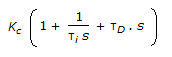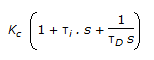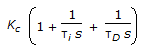# Chemical Engineering - Process Control and Instrumentation

### Exercise :: Process Control and Instrumentation - Section 1

31.

Which of the following measurements can be made by the measurement of emf ?

 A. pH value B. Degree of hydrolysis C. Composition of complex ions D. All (a), (b) & (c)

Answer: Option D

Explanation:

No answer description available for this question. Let us discuss.

32.

A magnetic flowmeter is

 A. based on the principle of Faraday's law. B. capable of measuring the flow rate of slurries and electrolytes. C. based on the linear relationship between the fluid flow rate and the induced voltage. D. all (a), (b) and (c).

Answer: Option D

Explanation:

No answer description available for this question. Let us discuss.

33.

Zirconia probe is used for the continuous measurment of __________ flue gases going out of the furnace.

 A. oxygen in B. carbon dioxide in C. carbon monoxide in D. temperature of

Answer: Option A

Explanation:

No answer description available for this question. Let us discuss.

34.

Strain gage pressure transducers are used to measure __________ pressures.

 A. gage as well as vacuum B. absolute as well as differential C. both (a) and (b) D. neither (a) nor (b)

Answer: Option C

Explanation:

No answer description available for this question. Let us discuss.

35.

The transfer function for a PID controller is (where, τ i is the integral (reset) time and τD is the derivative time.)

 A. Kc(1 + τis + τD ). s B.C.D.Answer: Option B

Explanation:

No answer description available for this question. Let us discuss.

#### Current Affairs 2021

Interview Questions and Answers